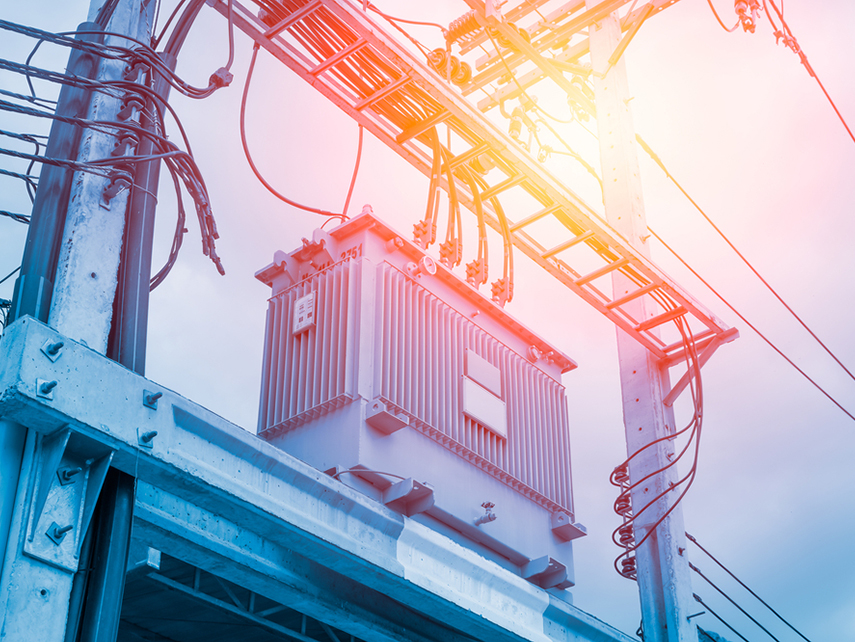# What is transformer rating? Types of transformer rating?

Table of content :

1. What is transformer rating?

2. Rated capacity of the transformer.

4. Meaning of power factor and energy transfer efficiency of the machine

5. Transformer working modes

## 1. What is transformer rating?

Transformer rating is the amount of electricity that the machine can convert for the load behind it. Since a transformer has only the function of transmitting and distributing power, not an energy conversion device such as an electric motor, the rated capacity of the transformer is in units of kVA, not kW. When transmitting the power, the machine's power has 2 components: reactive power kVA and active power kW.

## 2. Rated power of the transformer.

When the transformer is produced, it operates on a continuous and long-term basis, usually from 17 to more than 20 years. Manufacturers calculate the machine to work with the long-term mode without causing problems called the rated mode. Then the machine capacity corresponding to that mode is the rated power corresponding to that capacity, the specifications such as voltage U, current I, frequency f are rated.

Rated power is the total (apparent) power specified by the manufacturer in the machine profile.

This transformer can be continuously loaded (S = S rated) when the voltage is rated U, rated frequency f, and the cooling condition is rated and then the transformer's life will be equal about 20 years.

1. For 1-phase and two-phase transformer, the rated power is the winding capacity, and the total power per coil.

2.With a capacity of a 3-phase transformer, the manufacturer shall follow the following types:

• 100/100/100 is the type of which the capacity of each coil is equal to the rated power.
• 100/100 / 66,7 is the type with the capacity of the two windings equal to the rated power and the capacity of the third winding equal to 66,7% of the rated power.

3 For autotransformers, the rated power is either primary or secondary terminals which are automatically related, this power is also called the throughput.

## 3. Rated power transformer calculation formula:

It is known that the capacity of the single machine is kVA: total reactive power and active power are total power. The transformers mainly use reactive power to convert the voltage with the formula S = U.I for 1-phase machines and S = U.I with 3-phase machines.

Active power is calculated using the formula P = U.I.cosϕ (kW)

Reactive power, calculated using the formula Q = U.I.sinϕ (kVA)

Then the actual capacity of the machine includes the lost power of the machine and has the formula:

P = S.cosϕ

Inside:

S = U.I

P - units of W or KW

S - units VA or KVA

U - unit voltage difference V (Volt)

I - unit current A (ampere)

ϕ is the phase difference angle between the current and the voltage across the power-consuming equipment

Cosϕ - called power factor.

## 4. Meaning of power factor and energy transfer efficiency of the machine

When the transformer is working, it will receive energy (active power) from grid P1. Through the conversion process + iron and copper losses ... The rest is the capacity P2 provides to the load.

Efficiency Ƞ = P2 / (P2 + total loss)

With P2 = S.cosϕ (S = P - active power + Q - reactive power)

Transformer Q reactive power does not produce useful work, but is necessary for the energy conversion of transformers with units of VAR or kVAr. Reactive power Q (kVAr) is responsible for magnetizing the steel core in the machine to transfer power from primary to secondary. Reactive power Q is considered unjust power. Therefore, to increase the transformer's total capacity, we must increase the coefficients so that roughly = 1. Thus, we will improve the performance of the machine.

## 5. Transformer working modes

When the transformer is loaded, the change in the load current and the change in the power source will lead to a change in the secondary voltage leading to the machine working in 3 modes: overload, rated and under download.

In two cases, the machine is running under load or overloaded, which adversely affects the operation of the transformer. In the under-load or no-load mode, the cosϕ coefficient is small, so during operation, avoid the transformer idling or under load because the cosϕ factor is too small will adversely affect the grid.

In the case the machine is overloaded for a long time, it will heat up the device and affect the equipment and transformer insulation. As a rule, when the grid voltage changes by 5% of the rated voltage are also considered rated.

To help you choose the optimal transformer for your project, we provide a national regulatory standard for transformer-rated capacity ranges. The power levels of the machine are according to TCVN 6306-1: 2006 or IEC 60076-1: 2000 for reference by readers. If you have a design need according to your own standards, you can contact us for the best support.

.(+84) 0913.006.538
Skype, Telegram, Wechat ...